﻿ Effect of Viscosity on Nonlinear Tempering for Anomalous Diffusion of Viscous Particle: A Subdiffusive CasePublications are Open
Access in this journal
Article Versions
Export Article
• Normal Style
• MLA Style
• APA Style
• Chicago Style
Research Article
Open Access Peer-reviewed

### Effect of Viscosity on Nonlinear Tempering for Anomalous Diffusion of Viscous Particle: A Subdiffusive Case

Norodin A. Rangaig , Caironesa T. Pada, Vernie C. Convicto
International Journal of Physics. 2018, 6(1), 26-29. DOI: 10.12691/ijp-6-1-5
Published online: January 29, 2018

### Abstract

In this study, we introduced another parameter on nonlinear particle interaction into sub- diffusive transport involving nonlinear effects such as adhesion, volume filling, etc. We also introduce an additional variable which is the effect of viscosity on the nonlinear escape rate of particle which affects the resulting integral escape rate. This paper focuses only on the in- vestigation of the effect of the added variable on the total escape rate. Lastly, we can see the importance of this study when dealing viscous macroparticles.

### 1. Introduction

Anomalous diffusion can be found in a wide diversity of systems and is characterized by a sublinear growth of the mean square displacement (MSD) of the form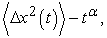where α is known as anomalous exponent and for subdiffusive case, 0 < α < 1. Subdiffusive regime can be observed in various area like in stochastic processes 1, application in financial market 2, modelling different physical phenomena 3, 4, application in the biological systems like the transport of lipids 5, and modelling the transport of particles in dendrites 6. Continuous time random walk (CTRW) is the standard model for the subdiffusive transport (see 7, 8, 9, 10 and the references therein) which is associated with waiting times ditributions with finite mean that leads to fractional equations in the diffusive limit 11, 12. In 12, it has been shown that the fractional equation is not structurally stable with respect to spatial fluctuation in the anomalous exponent. Due to this breakdown, different models have been proposed which tempers the anomalous waiting times of CTRW and such way is the nonlinear interactions which redistribute the particles when the local concentration grows too high 13.

In this paper, we intoduce a modified nonlinear escape rate which acts as tempering to the anoma- lous transport and is associated with two independent variables. This study is organized as follows: In section 2, we give some brief review on CTRW, specifically, the non-Markovian random walk which is rated as nonlinear random walk model. Within section 2, nonlinear interaction and structures of the particles were discussed. In section 3, we discuss the results, analysis, and findings of this paper and compare it to some previous articles.

### 2. Non-Markovian Random Walk

Consider a particle performing a 1-dimensional random walk making an instantaneous jump from location x to another, with jumplength l and waiting time T. In this study, we consider the jumps on right and left as independent and separate random variables and also associated with different waiting times. We denote the waiting time of the jump on right as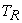and on left as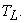We can have the actual waiting time given by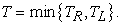For different waiting timesand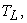we have the corresponding probability distribution functions (PDF)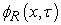and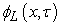respectively. τ is the representation of time a particle has remained at location x before jumping to another position. In general, this process is non-Markovian and otherwise if T is drawn from an exponential distribution.

Escape rate can be defined as an instantaneous transition probability from one state to another. According to Falconer et. al (2015) 14, a key feature of these escape rates is that they are dependent on the residence time parameter τ making the process non-Markovian. Let the rate of transition from x (x + l) denoted by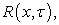and from x (x l) as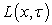defined respectively as: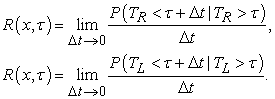If the escape rate is constant with respect to τ, we have the classical Markov process. However, the above form implies a lower probability of escaping for large value of τ and expreriencing a long trapping events, thus, making the non-Markov random walk model protected from any influence of external factors. In the next subsections, we will describe a particle random walk model where the density ρ and viscosity ν may affect the rate of escape of particles.

2.1. Nonlinear Interaction

In this part, we will modify again the modified escape rates in 14. Our modification gives more generalization which allows us to observe several macroscopic nonlinear effects. This introduced process is also an stochastic process which may act independently on the non-Markov trapping and allows the density and viscosity to play a role in the transport of particle even during long trapping events. We extend our modification as follows: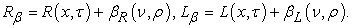(1)

where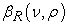and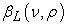are the additional nonlinear escape rates. If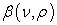is separable without affecting the nonlinear dependence on x and t, we can say that the probability due to this nonlinear term is independent from the anomalous trapping. On a given time interval, we have the probability as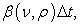where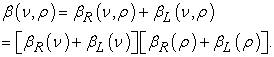We now analyze the dependence of these nonlinear escape rates due to the added variables that leads to different qualitative macroscopic effects. Volume filling effect describes a model where diffusing particle have non-zero volume, and occupying space they may prevent other particle to do the same 15. Analytically, this phenomenon reduces the molecular constriction of the particles, hence reduces the viscosity and we have the good choices for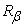and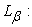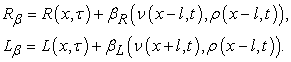The same with adhesion effect, where we increase the constriction of particles, hence increasing the bonding of the particles. We have the following choices forand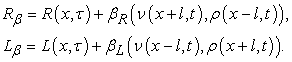Due to these analyses, we deduce the dependency of the escape rate on the density and viscosity of the particle. In general, we can have the dependence upon the local gradient as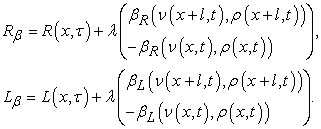In the subdiffusive case, we can deduce that the nonlinear term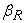and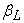have the effect of tempering factors.

2.2. Structured Density and Viscosity of Particles

In order to describe the evolution of the introduced non-Markovian process, let us consider a density number distribution (DND) of the form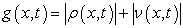(2)

which generalizes the effects and contribution of both density and viscosity. Now, we can have the balance equation for γ given by,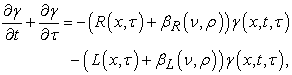(3)

and we aim to identify the unstructured (DND) of the form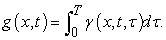(4)

Before we proceed, we should define some key features from the random walk model which is an effective tool in this study. Let the probability density functions (PDFs) for survival function be denoted as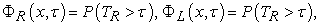and the probability for a particle to remain at some position at given time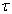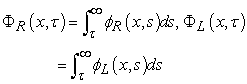(5)

where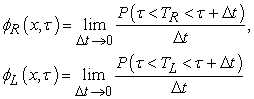(6)

are the PDFs for the waiting times for jumps to the right and to the left, respectively. From the definition of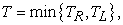we can write the total survival PDF as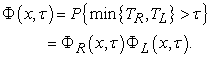In the next section, we will discuss the resulting total escape rate with the effect of both density and viscosity.

### 3. Results and Discussions

In equation (3), observe that it is written in terms of a combination of the two escape rate. The initial condition at t = 0 may be written as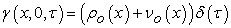and for the boundary condition where there is no residence time (τ = 0) is: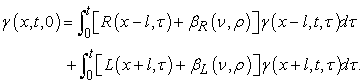Integrating over τ, we can obtain the total escape rate of the right and left, respectively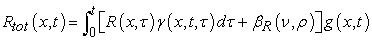(7)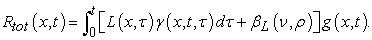(8)

To fully obtain the total escape rate, let us solve equation (3) using characteristic method for τ < t, one can get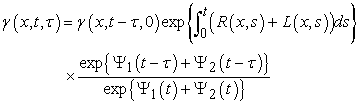(9)

where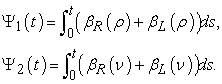Let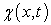denotes the integral rate of arrival of the particle, such that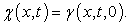(10)

Using equation (9), we can immediately write equation (10) as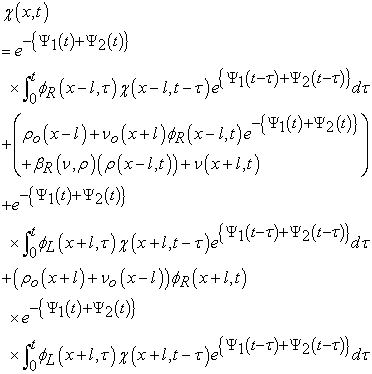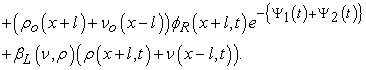We can see the contribution of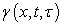due to the singularity at the initial condition which is on the second term. Substituting equation (9) for the unstructured DND in equation (4), we have: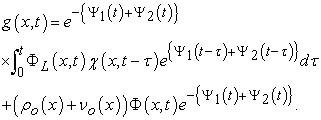(11)

Using equation (9), we can finally obtain the total integral escape rates as: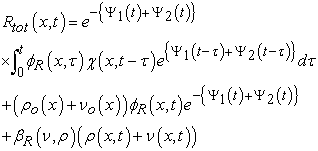(12)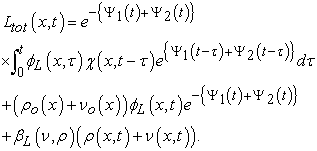(13)

The obtained result of the total escape rates shows a generalized form where both density and viscosity play a versatile role in tempering anomalous subdiffuion. In constrast to some previous studies 14, 16, 17, where the density plays a very important role in modelling physical behavior, our result extends the application on viscous particle, especially in modelling plasma uids where viscosity and density are the main factors of its transport.

### 4. Conclusions

In this study, we investigate the effect of the viscosity on the total escape rate of the particle undergoing subdiffusion. Upon introducing the nonlinear term on the existing escape rate, which is dependent on the viscosity and density, we then posited that we should generalized the effect of density and viscosity and introduce a new density number distribution (2). We also obtained the structure of the particle given by equation (11), which shows spatial and temporal dependency. Analysis of the main result shows that the introduced variable, which is the viscosty, generalizes the existing escape rate of previous literature. Furthermore, if we have the condition ρ >> ν, the total escape rate in (12 and 13) boils down to the existing escape rate in 14, 16, thus leaving only the density to take effect on the transport. Lastly, modelling the transport of viscous particle can be done using the obtained total escape rate upon obtaining the master equation for both density and viscosity.

### Acknowledgements

The authors would like to thank the Department of Physics, Mindanao State University-Main Campus for the support extended on this paper.

None.

### References

  S. Bochner, Diffusion equation and stochastic processes, Proceedings of the National Academy of Sciences, vol. 35, no. 7, pp. 368370, 1949. In article View Article  H. Gu, J.-R. Liang, and Y.-X. Zhang, On a time-changed geometric Brownian motion and its application in financial market, Acta Physica Polonica B, vol. 43, no. 8, pp. 16671681, 2012. In article View Article  J. Janczura and A. Wyomanska, Anomalous diffusion models: different types of subordinator distribution, Acta Physica Polonica B, vol. 43, no. 5, pp. 10011016, 2012. In article View Article  C. Song, T. Koren, P. Wang, and A. Barabasi, Modelling the scaling properties of human mobility, Nature Physics, vol. 6, no. 10, pp. 818823, 2010. In article View Article  M.J Saxton, Anomalous Subdiffusion in Flouresence Photobleaching Recovery: A Monte Carlo Study, Biophysical Journal, 1985. In article View Article  S. Fedotov, and V. Mendez, Non-Markovian Model for Transport and Reaction of Particles in Spiny Dendrites, Phys. Rev. Lett., 101: 218102, 2008. In article View Article  PubMed  D. Kleinhans and R. Friedrich, Continuous-time random walks: simulation of continuous tra- jectories, Physical Review E, vol. 76, no. 6, Article ID 061102, 2007. In article View Article  M.Magdziarz, Langevin picture of subdiffusion with infinitely divisiblewaiting times, Journal of Statistical Physics, vol. 135, no. 4, pp. 763772, 2009. In article View Article  M. Magdziarz, Stochastic representation of subdiffusion processes with time-dependent drift, Stochastic Processes and Their Applications, vol. 119, no. 10, pp. 32383252, 2009. In article View Article  E. Scalas, The application of continuous-time randomwalks in finance and economics, Physica A: StatisticalMechanics and its Applications, vol. 362, no. 2, pp. 225239, 2006. In article View Article  M. Magdziarz, A. Weron, and K. Weron, Fractional Fokker- Planck dynamics: stochastic representation and computer simulation, Physical Review EStatistical, Nonlinear, and Soft Matter Physics, vol. 75, no. 1, Article ID016708, 2007. In article View Article  S. Fedotov, and S. Falconer, Subdiffusive Master Equation with Space-Dependent Anomalous Exponent and Structural Instability, Phys. Rev. E, 85(3):1-6, 2012. In article View Article  PubMed  P. Straka and S. Fedotov, Transport Equation for Subdiffusion with Nonlinear Particle Inter- action, arXiv preprint: 1404.6869, 2014. In article View Article  S. Falconer, A. Al-Sabbagh, and S. Fedotov, Nonlinear Tempering of Subdiffusion with Chemo- taxis, 2015. In article View Article  T. Hillen, and K. Painter, Global Existence foe a Parabolic Chemotaxis Model with Prevention of Overcrowding. Adv. in Appl. Math., 26(4): pp. 280-301, 2001. In article View Article  S. Fedotov, and S. Falconer, Random Death Process for the Regularization of Subdiffusive fractional Equation, Phys. Rev. E, 87:052139, 2013. In article View Article  PubMed  S. Fedotov, and S. Falconer, Nonlinear Degradation-enhanced Transport of Morphogens Per- forming Subdiffusion, Phys. Rev. E, 89:012107, 2014. In article View Article  PubMedThis work is licensed under a Creative Commons Attribution 4.0 International License. To view a copy of this license, visit http://creativecommons.org/licenses/by/4.0/